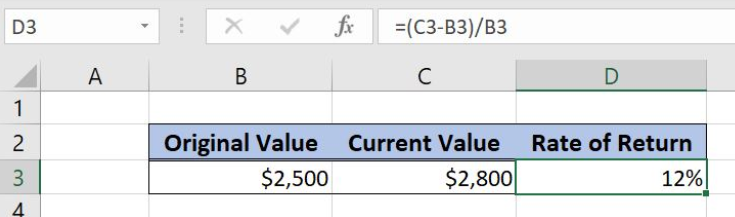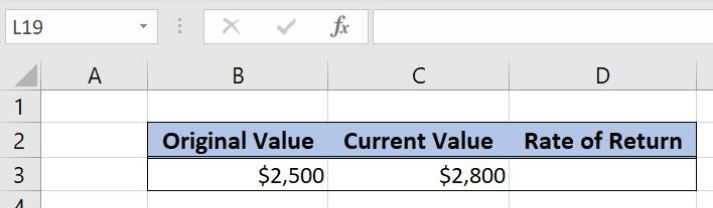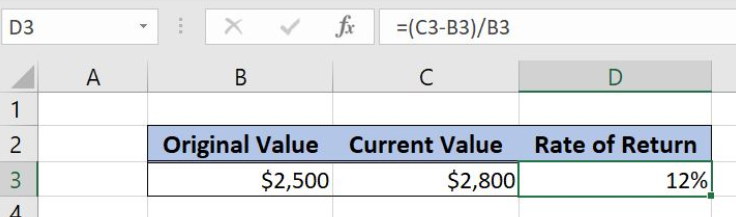Get instant live expert help with Excel or Google Sheets“My Excelchat expert helped me in less than 20 minutes, saving me what would have been 5 hours of work!”

#### Post your problem and you’ll get expert help in seconds.

Your message must be at least 40 characters
Our professional experts are available now. Your privacy is guaranteed.

# Discover How to Find the Rate of Return in Excel

Excel allows a user to calculate profit margin percentage from a sale price and cost, using the simple formula. The profit margin is calculated as a rate between price and cost difference and price. This step by step tutorial will assist all levels of Excel users in getting a profit margin percentage.Figure 1. The result of the formula

## Syntax of the Formula

The generic formula is:

`=(current_value - original_value)/original_value`

The parameters of the formula are:

• current_value – the current value of a purchase
• original_value – the original value of a purchase.

## Setting up Our Data for the Example

Let’s look at the structure of the data we will use. In B3, we have the original value and in C3 we have current value after some period. In the cell D3, we want to calculate a rate of return.Figure 2. Data that we will use in the example

## Calculating the Profit Margin Percentage

In our example, the original value is \$2,500 (B3) and the current value is \$2,800 (C3) Based on these two values, we want to calculate the rate of return in the cell D3.

Formula:

`=(C3 - B3)/B3`

The parameter current_value is C3 and the original_value is B3.

To apply the formula, we need to follow these steps:

• Select cell D3 and click on it
• Insert the formula: `=(C3-B3)/B3`
• Press enter.Figure 3. Calculating the rate of return percentage

The result in the cell D3 is 12%, which is the rate of return for the above parameters.

Most of the time, the problem you will need to solve will be more complex than a simple application of a formula or function. If you want to save hours of research and frustration, try our live Excelchat service! Our Excel Experts are available 24/7 to answer any Excel question you may have. We guarantee a connection within 30 seconds and a customized solution within 20 minutes.